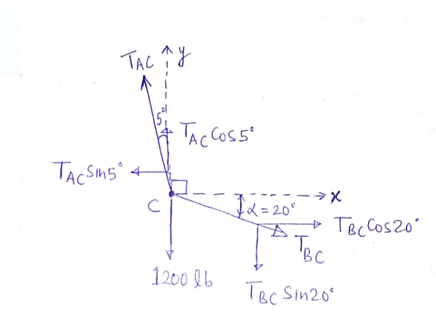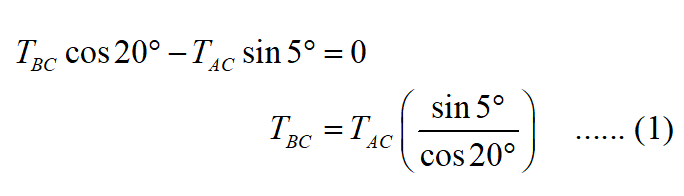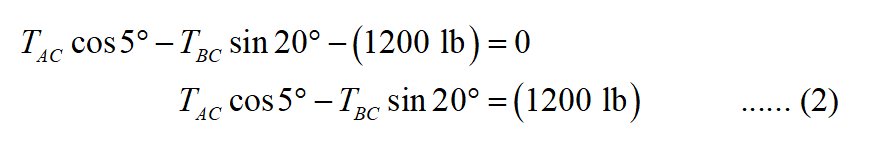# Knowing that α = 20∘, determine the tension (a) in cable AC, (b) in rope BC.

Question-AnswerCategory: Engineering MechanicsKnowing that α = 20∘, determine the tension (a) in cable AC, (b) in rope BC.

Knowing that α20, determine the tension (a) in cable AC, (b) in rope BC.(a)
Consider the above-given diagram and draw a free body diagram of point C,Now apply the force equilibrium in the x-direction,Now apply the force equilibrium in the y-direction,From equations (1) and (2),Therefore, the tension in cable AC is 1244.2 lb.

(b)
From equation (1),Therefore, the tension in rope BC is 115.4 lb